﻿

Congruences for (2, 3)-regular partition with designated summands

Abstract

Let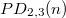$PD_{2, 3}(n)$ count the number of partitions of$n$ with designated summands in which parts are not multiples of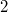$2$ or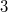$3$. In this work, we establish congruences modulo powers of 2 and 3 for$PD_{2, 3}(n)$. For example, for each \quad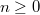$n\ge0$ and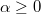$\alpha\geq0$ \quad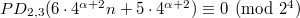$PD_{2, 3}(6\cdot4^{\alpha+2}n+5\cdot4^{\alpha+2})\equiv 0 \pmod{2^4}$ and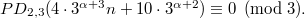$PD_{2, 3}(4\cdot3^{\alpha+3}n+10\cdot3^{\alpha+2})\equiv 0 \pmod{3}.$

DOI Code: 10.1285/i15900932v36n2p99

Keywords: Designated summands; Congruences; Theta functions; Dissections

Full Text: PDF